Anúncio

# arithmetic sequence and series.pptx

28 de Mar de 2023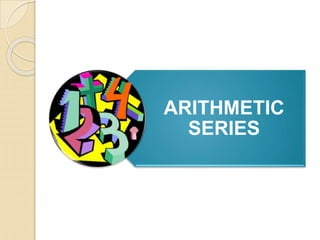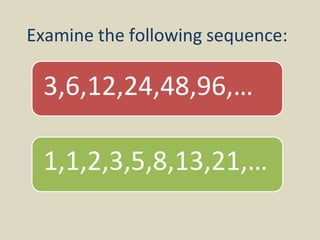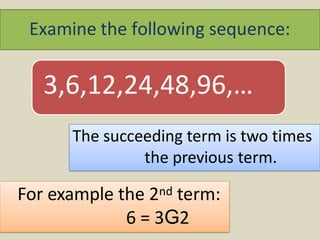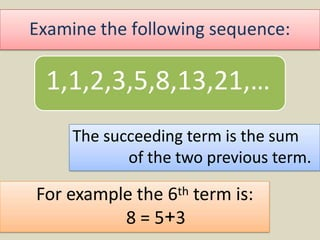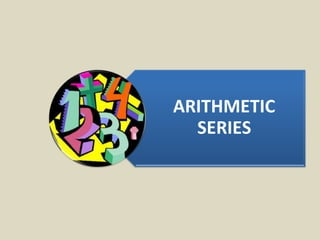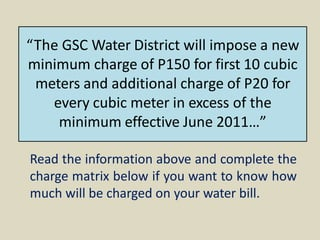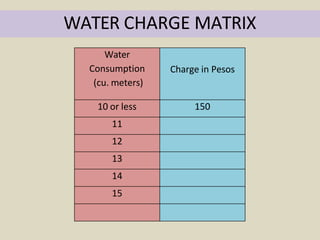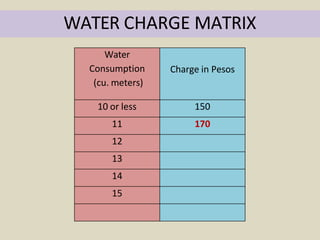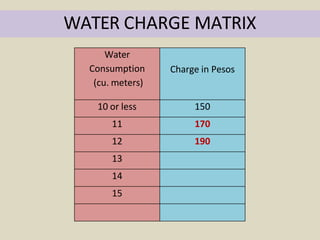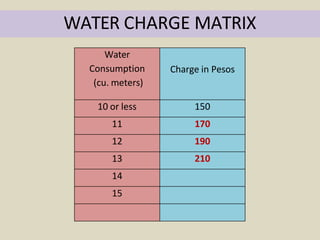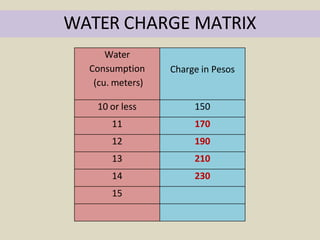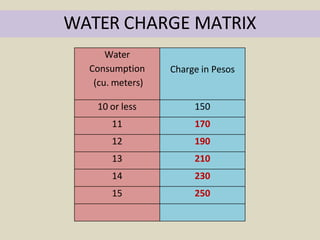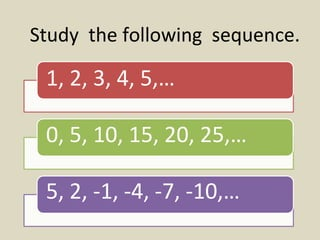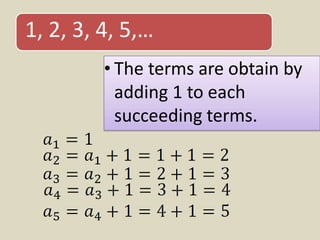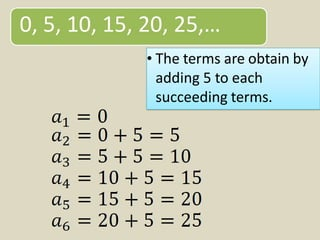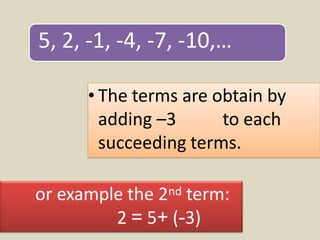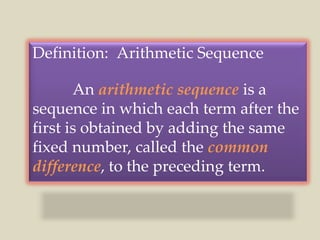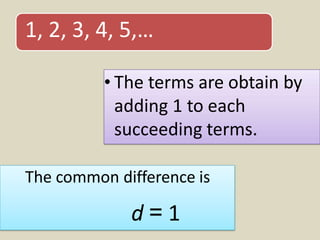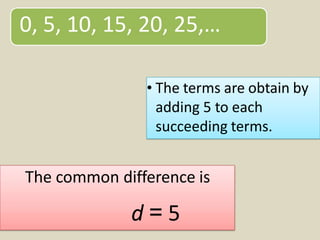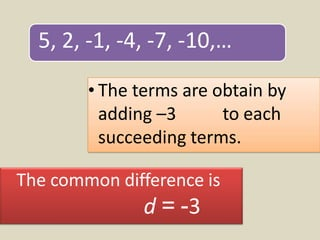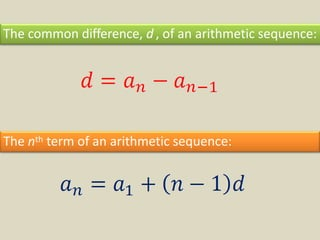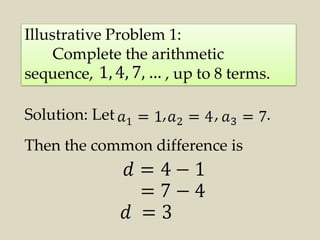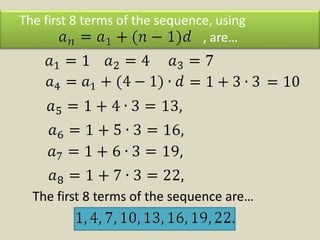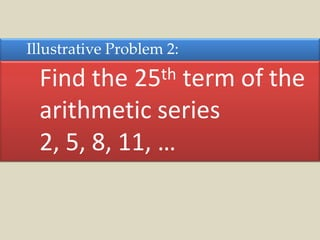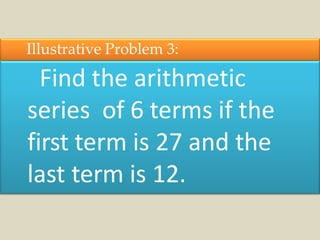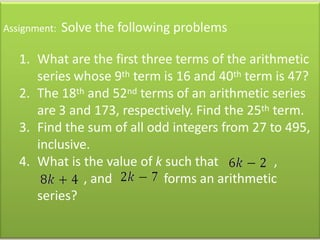1 de 27
Anúncio

### arithmetic sequence and series.pptx

1. ARITHMETIC SERIES
2. Examine the following sequence: 3,6,12,24,48,96,… 1,1,2,3,5,8,13,21,…
3. Examine the following sequence: 3,6,12,24,48,96,… The succeeding term is two times the previous term. For example the 2nd term: 6 = 3G2
4. Examine the following sequence: The succeeding term is the sum of the two previous term. For example the 6th term is: 8 = 5+3 1,1,2,3,5,8,13,21,…
5. ARITHMETIC SERIES
6. “The GSC Water District will impose a new minimum charge of P150 for first 10 cubic meters and additional charge of P20 for every cubic meter in excess of the minimum effective June 2011…” Read the information above and complete the charge matrix below if you want to know how much will be charged on your water bill.
7. WATER CHARGE MATRIX Water Consumption (cu. meters) Charge in Pesos 10 or less 150 11 12 13 14 15
8. WATER CHARGE MATRIX Water Consumption (cu. meters) Charge in Pesos 10 or less 150 11 170 12 13 14 15
9. WATER CHARGE MATRIX Water Consumption (cu. meters) Charge in Pesos 10 or less 150 11 170 12 190 13 14 15
10. WATER CHARGE MATRIX Water Consumption (cu. meters) Charge in Pesos 10 or less 150 11 170 12 190 13 210 14 15
11. WATER CHARGE MATRIX Water Consumption (cu. meters) Charge in Pesos 10 or less 150 11 170 12 190 13 210 14 230 15
12. WATER CHARGE MATRIX Water Consumption (cu. meters) Charge in Pesos 10 or less 150 11 170 12 190 13 210 14 230 15 250
13. Study the following sequence. 1, 2, 3, 4, 5,… 0, 5, 10, 15, 20, 25,… 5, 2, -1, -4, -7, -10,…
14. • The terms are obtain by adding 1 to each succeeding terms. 1, 2, 3, 4, 5,…
15. • The terms are obtain by adding 5 to each succeeding terms. 0, 5, 10, 15, 20, 25,…
16. • The terms are obtain by adding –3 to each succeeding terms. 5, 2, -1, -4, -7, -10,… or example the 2nd term: 2 = 5+ (-3)
17. Definition: Arithmetic Sequence An arithmetic sequence is a sequence in which each term after the first is obtained by adding the same fixed number, called the common difference, to the preceding term.
18. • The terms are obtain by adding 1 to each succeeding terms. 1, 2, 3, 4, 5,… The common difference is d = 1
19. • The terms are obtain by adding 5 to each succeeding terms. 0, 5, 10, 15, 20, 25,… The common difference is d = 5
20. • The terms are obtain by adding –3 to each succeeding terms. 5, 2, -1, -4, -7, -10,… The common difference is d = -3
21. The nth term of an arithmetic sequence: The common difference, d , of an arithmetic sequence:
22. Illustrative Problem 1: Complete the arithmetic sequence, , up to 8 terms. Solution: Let , , . Then the common difference is
23. The first 8 terms of the sequence, using , are… The first 8 terms of the sequence are…
24. Illustrative Problem 2: Find the 25th term of the arithmetic series 2, 5, 8, 11, …
25. Illustrative Problem 3: Find the arithmetic series of 6 terms if the first term is 27 and the last term is 12.
26. Assignment: Solve the following problems 1. What are the first three terms of the arithmetic series whose 9th term is 16 and 40th term is 47? 2. The 18th and 52nd terms of an arithmetic series are 3 and 173, respectively. Find the 25th term. 3. Find the sum of all odd integers from 27 to 495, inclusive. 4. What is the value of k such that , , and forms an arithmetic series?
27. ARITHMETIC SERIES
Anúncio